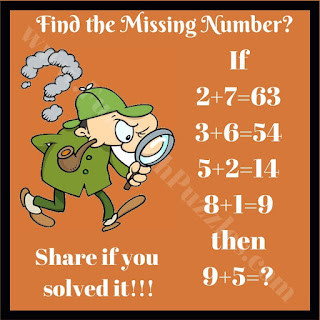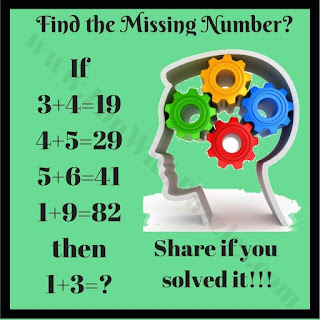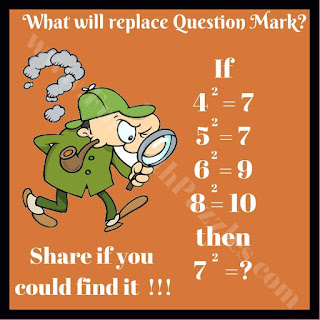## Saturday, December 7, 2019

This is the second part of Mathematics Logic Brain Cracking Problems. These are the mind-challenging puzzle questions which will not only test your Mathematical skills but also logical reasoning. In these picture puzzles, you are presented with some equations which are Mathematical incorrect but these equations contain some logical pattern which makes these equations logical correct. Your challenge is to find this logical pattern and then solve the last equation.6. Can you solve this Maths Equation?7. Can you solve this Math Tricky Riddle?8. Can you solve this Mathematical Puzzle?9. Can you solve this Tough Maths Puzzle?10. Can you solve this tricky Brain Puzzle?

These Maths Logical Questions tested your Mathematical ability and logical thinking. Do checkout below listed fun puzzles and brain teasers to test your brain.

## List of Fun Puzzles and Brain Teasers

1.1.Nice to know that your liked these maths logical questions. There are so many similar #puzzles on this website. Solve these puzzles and keep posting your comments.

2.If
3+3=45
4+4=80
Then
6+6=?

1.Answer to this logical number puzzle is 6x6x5 = 180

3.12×12=9
23×23=16
34×34=?

4.